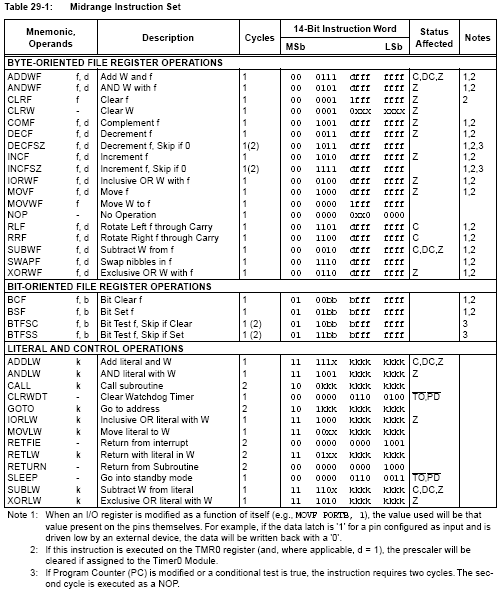PIC INSTRUCTIONS This discussion explains the instructions for the PIC12F629 and PIC16F628.  addlw number - adds a number (called a literal -  00 to FF) to the number in the working register. addwf FileReg, f - adds the number in the working register to the number in a file (FileReg) and puts the result in the file. addwf FileReg, w - adds the number in the working register to the number in a file (FileReg) and puts the result back into the working register, leaving the file register unchanged. andlw number - ANDs a number to the number in the working register, leaving the result in the working reg. andwf FileReg, f - ANDs the number in the working register to the number in a file (FileReg) and puts the result in the file register bcf FileReg, bit - clears a bit in a file (FileReg), i.e. makes the bit 0 bsf FileReg, bit - sets a bit in a file (FileReg), i.e. makes the bit 1 btfsc FileReg, bit - tests a bit in a file (FileReg) and skips the next instruction if the result is clear (i.e. if that bit is 0). btfss FileReg, bit - tests a bit in a file (FileReg) and skips the next instruction if the result is set (i.e. if that bit is 1). call label - makes the chip call any label, after which it will return to where it left off if the sub-routine contains retlw 00. clrf File - clears (makes 0) the number in a file (FileReg). clrw - clears the working register. clrwdt - clears the watchdog timer. comf FileReg, f - complements (inverts - ones become zeroes, zeroes become ones) the number in a file (FileReg), leaving the result in the file register. decf FileReg, f - decrements (subtracts one from) a file (FileReg) and puts the result in the file register. decfsz FileReg, f - decrements a file (FileReg) and if the result is zero it skips the next instruction. The result is put in the file. goto Label - makes the chip go to a label in the program. incf FileReg, f - increments (adds one to) a file (FileReg) and puts the result in the file. incfsz FileReg, f - increments a file(FileReg) and if the result is zero it skips the next instruction. The result is put in the file. iorlw number - inclusive ORs a number with the number in the working register. iorwf FileReg, f - inclusive ORs the number in the working register with the number in a file(FileReg) and puts the result in the file. movfw FileReg or movf FileReg, w - moves (copies) the number in a file(FileReg) in the working register movlw number - puts a number into the working register. movwf FileReg - moves (copies) the number in the working register into a file (FileReg). nop - this stands for : no operation, in other words - do nothing, (it is used to create a delay) option_reg - takes the number in the working register and moves it into the option register. retfie - (NOT FOR PIC16C5X SERIES) - returns from a subroutine and enables the Global Interrupt Enable bit. retlw number - returns from a subroutine with a number (literal) (from 00 to FF) in the working register. rlf FileReg, f - rotates the bits in a file (FileReg) to the left, putting the result in the file. rrf FileReg, f - rotates the bits in a file(FileReg) to the right, putting the result in the file. sleep - sends the PIC to sleep, a lower power consumption mode. sublw number - subtracts the number in the working register from a literal. To subtract 1 from W, use: addlw   255 subwf FileReg, f - subtracts the number in the working register from the number in a file (FileReg) and puts the result in the file. swapf FileReg, f - swaps the two halves of the 8 bit binary number in a file (FileReg), leaving the result in the file. movwf    tris PORTA  or     movwf    trisPORTB    or            movwf    trisio      for PIC12F629 - moves the value in w to the tris file. xorlw number - exclusive ORs a literal with the number in the working register. xorwf FileReg, f - exclusive ORs the number in the working register with the number in a file (FileReg) and puts the result in the file.